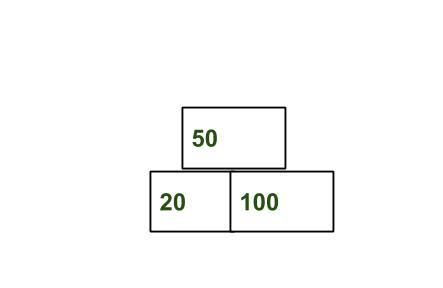# Find maximum height pyramid from the given array of objects

Given n objects, with each object has width wi. We need to arrange them in a pyramidal way such that :

1. Total width of ith is less than (i + 1)th.
2. Total number of objects in the ith is less than (i + 1)th.The task is to find the maximum height that can be achieved from given objects.

Examples :

```Input : arr[] = {40, 100, 20, 30}
Output : 2
Top level : 30.
Lower (or bottom) level : 20, 40 and 100
Other possibility can be placing
20 on the top, and at second level any
other 4 objects. Another possibility is
to place 40 at top and other three at the
bottom.

Input : arr[] = {10, 20, 30, 50, 60, 70}
Output : 3```

The idea is to use greedy approach by placing the object with the lowest width at the top, the next object at the level right below and so on.
To find the maximum number of levels, sort the given array and try to form pyramid from top to bottom. Find the smallest element of array i.e first element of array after sorting, place it on the top. Then try to build levels below it with greater number of objects and greater width.

Below is the implementation of this approach:

// C++ program to find maximum height pyramid
// from the given object width.
#include<bits/stdc++.h>
using namespace std;

// Returns maximum number of pyramidcal levels
// n boxes of given widths.
int maxLevel(int boxes[], int n)
{
// Sort objects in increasing order of widths
sort(boxes, boxes + n);

int ans = 1; // Initialize result

// Total width of previous level and total
// number of objects in previous level
int prev_width = boxes;
int prev_count = 1;

// Number of object in current level.
int curr_count = 0;

// Width of current level.
int curr_width = 0;
for (int i=1; i<n; i++)
{
// Picking the object. So increase current
// width and number of object.
curr_width += boxes[i];
curr_count += 1;

// If current width and number of object
// are greater than previous.
if (curr_width > prev_width &&
curr_count > prev_count)
{
// Update previous width, number of
// object on previous level.
prev_width = curr_width;
prev_count = curr_count;

// Reset width of current level, number
// of object on current level.
curr_count = 0;
curr_width = 0;

// Increment number of level.
ans++;
}
}

return ans;
}

// Driver Program
int main()
{
int boxes[] = { 20, 30, 40, 100};
int n = sizeof(boxes)/sizeof(boxes);
cout << maxLevel(boxes, n) << endl;
return 0;
}

Output :

```3
```

Time Complexity : O(n log n)#### More Articles of M Mounika:

Name Views Likes
C++ Segmented Sieve (Print Primes In a Range) 162 0
C++ Sieve Of Erastosthenes 135 0
C++ Gold Mine Problem 295 0
C++ Merge K Sorted Arrays 117 0
C++ K Centers Problem 240 0
C++ Find Nth Catalan Number 311 0
C++ Inplace Rotate square matrix by 90 degrees 285 0
C++ Find Non Repeating Elements in Array 87 0
C++ Merge Two Binary Trees 121 0
C++ Sum of Numbers From Root To Leaf Paths 89 0
C++ Meta Strings 91 0
C++ Flood Fill Algorithm 402 0
C++ smallest substring with maximum distinct characters 200 0
C++ Smallest window with all characters in string 94 0
C++ Minimum Removal of Characters from string to make its permutation as palindrome 87 0
C++ Minimum characters added at front of string in palindrome conversion 69 0
C++ Number of Bracket Reversals needed to make expression Balanced 72 0
C++ String to Palindrome with Append Function 83 0
C++ WildCard pattern matching 76 0
C++ Anagram substring Search 72 0
C++ Manachars Algorithm 74 0
C++ Search String in Grid 83 0
C++ String Matching(Z Algorithm) 67 0
C++ String Matching(Naive Algorithm) 113 0
C++ String Matching(KMP Algorithm) 140 0
C++ Remove Duplicates From String 110 0
C++ Basics of String Manipulation 85 1
C++ Disjoint Data Structure Cycle Detection 87 0
C++ Problem On Disjoint Data Structures 95 0
C++ Disjoint Data Structures Part3 79 0
Disjoint Data Structures Part2 90 0
Disjoint Data Structures 93 1
C++ Segment Trees 321 2
C++ Trie Cost of Data 290 1
C++ Trie Datastructure 279 1
C++ Greedy Approach Minimum number of coins 526 0
C++ Greedy Approach Maximum height Pyramid 329 1
C++ Greedy Approach String lexicographically largest subsequence 247 0
C++ Greedy Approach Lexicographically largest subsequence 364 0
C++ Greedy Approach Prims MST 398 1
C++ Greedy Approach Krushkals MST 458 1
C++ Greedy Approach N-array maximum sum 334 1
C++ Greedy Approach Policemen Catch Thieves 563 1
C++ Greedy Approach Maximum product Subset 546 1
C++ Greedy Approach Minimum Product Subset 349 1
C++ Greedy Approach Fractional Knapsack 737 1
C++ Greedy Approach-Activity Selection Problem 745 1
C++ Greedy Approach-Egyptian Fractions 640 0
C++ Greedy Approach-Huffman Codes 1031 1
C++ Introduction to Greedy Approach 955 2